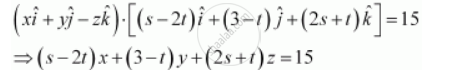Share

# Find the Cartesian Equation of the Following Planes: Vecr.[(S-2t)Hati + (3 - T)Hatj + (2s + T)Hatk] = 15 - CBSE (Science) Class 12 - Mathematics

ConceptVector and Cartesian Equation of a Plane

#### Question

Find the Cartesian equation of the following planes:

vecr.[(s-2t)hati + (3 - t)hatj + (2s + t)hatk] = 15

#### Solution

vecr.[(s-2t)hati + (3 - t)hatj + (2s + t)hatk] = 15  ....(1)

For any arbitrary point P (xyz) on the plane, position vector vecr is given by,

vecr = xhati + yhatj - zhatk

Substituting the value of hatr in equation (1), we obtainThis is the Cartesian equation of the given plane.

Is there an error in this question or solution?

#### APPEARS IN

NCERT Solution for Mathematics Textbook for Class 12 (2018 to Current)
Chapter 11: Three Dimensional Geometry
Q: 3.3 | Page no. 493

#### Video TutorialsVIEW ALL 

Solution Find the Cartesian Equation of the Following Planes: Vecr.[(S-2t)Hati + (3 - T)Hatj + (2s + T)Hatk] = 15 Concept: Vector and Cartesian Equation of a Plane.
S